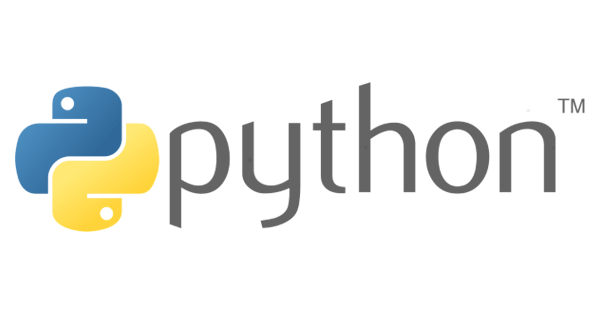# Python 基礎語法Python 的基礎語法說明，包含命名規則、註解方式、輸出、取得使用者輸入和命令列引數，還有與其它程式語言較大差異的使用縮排代替大括號，以及結尾可不加分號。

## 命名規則

• 與大多數程式語言一樣，可包含英文字母、數字與下底線，但不能以數字開頭。
• 英文字母區分大寫小。

## 縮排代替 {} 大括號

Python 使用縮排 (Indentation) 來識別程式區塊，不同於其它程式語言使用 `{}` 大括號的方式，因此程式碼看起來會較整潔。

PHP：

``````<?php
\$number = 1;

while (\$number <= 50) {
\$sum += \$number;
\$i++;
}``````

Python：

``````number = 1

while (number <= 50)
sum += number
i++``````

## 結尾語句不加分號

Python 與 JavaScript 相同，; 分號可加也可不加。

``````print('不加分號')
print('加分號');``````

## 註解

### 單行註解

``````#print('這是單行註解不會印出')
print('只有這行會印出')``````

### 多行註解

``````'''
print('這是連續三個單引號的多行註解，不會印出')
print('這是連續三個單引號的多行註解，不會印出')
'''

"""
print('這是連續三個雙引號的多行註解，不會印出')
print('這是連續三個雙引號的多行註解，不會印出')
"""

print('只有這行會印出')``````

## help() 用法

``````python3
Python 3.5.3 (default, Jan 19 2017, 14:11:04)
[GCC 6.3.0 20170124] on linux
``````# 查看看所有的內建函式
>>> dir(__builtins__)
[…, 'print', 'property', 'quit', 'range', 'repr', 'reversed', 'round', 'set', 'setattr', 'slice', 'sorted', 'staticmethod', 'str', 'sum', 'super', 'tuple', 'type', 'vars', 'zip']

# 查看 print() 內建函式的使用語法，不須加函式的 () 括號
>>> help(print)

# 查看字串類別的使用語法 (先使用 type() 查看字串的類別)
>>> type('s')
<class 'str'>
>>> help('str')

# 查看數值類別的使用語法 (可直接填入數值就會自動查看 int 類別)
>>> help(1)

# 查看 sys 模組的使用語法
>>> help('sys')

# 也可直接進入 help() 的提示字元查看，就可省略再輸入 help()
>>> help()

Welcome to Python 3.5's help utility!

... 以上省略 ...

help> print
help> str``````

## Output 輸出

``print(value, ..., sep=' ', end='\n', file=sys.stdout, flush=False)``
`print()` 內建函式參數表

`print()` value   要輸出的資料 可使用 , 逗號分隔指定多個 value
sep ' ' value 與 value 之間的分隔方式 一個 Space
end \n 資料最後的結束方式 \n 換行符號
file `sys.stdout` 將資料輸出至指定檔案
flush False True 立即將資料輸出至檔案 False 先將資料存至記憶體，待檔案關閉時才把資料輸出

``````#!/usr/bin/env python

# 輸出三個 value
print('A', 'B', 'C')
'''
A B C
'''

# value 與 value 使用 - 符號分隔
print('A', 'B', 'C', sep = '-')
'''
A-B-C
'''

# 資料最後的結束方式
print('A', 'B', 'C', end = '\n*****\n')
'''
A B C
*****
'''

# 將資料輸出至 data.txt 檔案
print('A', 'B', 'C', file = open('data.txt', 'w'))``````

``````python3 test.py
A B C
A-B-C
A B C
*****``````
``````cat data.txt
A B C``````

## Input 輸入

### 命令列引數

• ：執行的程式檔名。
•  ~ 之後：使用者所輸入的引數，以 Space 區隔多個引數。

``````#!/usr/bin/env python

import sys

print('sys.argv  :' + sys.argv)
print('sys.argv  :' + sys.argv)
print('sys.argv  :' + sys.argv)``````

``python3 test.py ABC 123``
``````sys.argv  : test.py
sys.argv  : ABC
sys.argv  : 123``````

### input() 取得使用者輸入

``````#!/usr/bin/env python

name = input('請輸入您的姓名：')
print('您好 ' + name + ' 先生 / 小姐！')``````

``python3 test.py``
``````請輸入您的姓名：Jacky